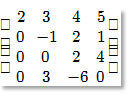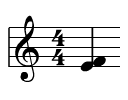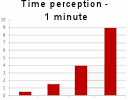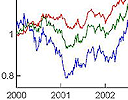# Archive for February, 2011

## IntMath Newsletter: quadratic, resources, pentatonic and MathJax

In this Newsletter:

1. Math tip - Quadratic formula by completing the square - easier method
2. Resource - Curriki (a curriculum wiki)
3. More funny GraphJam graphs
4. Friday math movie: pentatonic scale
5. MathJax - render math on the Web on all browsers
6. Final thought – things that count

## Free math resourcesHere is a list of math lessons and other resources that you may find useful.

## MathJax - render math on the Web on all browsersHere's another method for getting math onto a Web page - MathJax.

## Friday math movie: pentatonic scaleThe pentatonic scale is popular across all cultures. Some math is involved here!

## More funny GraphJam graphsHere are some more funny graphs courtesy of GraphJam.

## Quadratic formula by completing the square - easier methodHere's an easier way to derive the Quadratic Formula using completing the square.

## IntMath Newsletter - e Day, funny graphs, risk and matrices

In this Newsletter:

1. Math tip: Happy "e" Day
2. Funny graphs - from GraphJam
3. Math tip: Multiplying matrices
4. Friday math movie: Hans Rosling's 200 Countries, 200 Years, 4 Minutes
5. Math tip: Determining economic risk - Black Scholes formula
6. Friday math movie: Doodling in math class - binary trees
7. Final thought – security

## Determining economic risk - Black Scholes formulaThe Black Scholes Model is one of the many formulas used by the mathematical financial wizards to price options. How does it work?

## Multiplying matricesThis article points to 2 interactives that show how to multiply matrices.

## Full archive

See the blog sitemap for all previous articles.